MOBILE MATH – Math on the Go
www.mobilemath.ca

# 2 - Numeracy - Multiplying

Summary – Examples Covered

Screen Shot

2.1  – Numeracy – Multiplying - Positive Numbers - Intro

Smart Notebook Lesson 2.1

Learning Goal:

By the end of this lesson I should be able to MULTIPLY single digit positive numbers together WITHOUT the use of a calculator (technology).

Techniques:    Area Model (intro),             Number Model

Examples:

(a)                8 x 3

(b)               4 x 6

(c)                9 x 7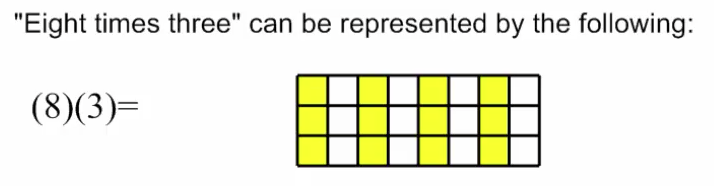2.2  – Numeracy – Multiplying - Area Model

Smart Notebook Lesson 2.2

Learning Goal:

By the end of this lesson I should be able to MULTIPLY single and double digit positive numbers together using an AREA MODEL; WITHOUT the use of a calculator (technology).

Techniques:    Area Model

Examples:

(a)                8 x 3

(b)               4 x 13

(c)                14 x 21

(d)               64 x 25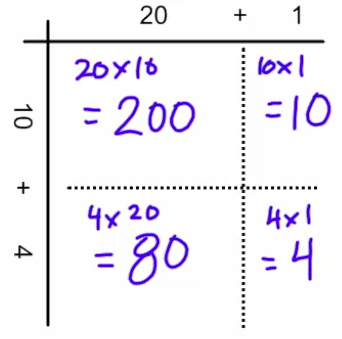2.3  – Numeracy – Multiplying - Area Model 2

Smart Notebook Lesson 2.3

Learning Goal:

By the end of this lesson I should be able to MULTIPLY double and triple digit positive numbers together using an AREA MODEL; WITHOUT the use of a calculator (technology).

Techniques:    Area Model

Examples:

(a)               4 x 13

(b)               38 x 25

(c)                274 x 462.4  – Numeracy – Multiplying - Distributive Property

Smart Notebook Lesson 2.4

Learning Goal:

By the end of this lesson I should be able to MULTIPLY single and double digit positive numbers together using the DISTRIBUTIVE PROPERTY of MULTIPLICATION; WITHOUT the use of a calculator (technology).

Techniques:    Area Model and Distributive Property

Examples:

(a)                4 x 13

(b)               8 x 21

(c)                6 x 75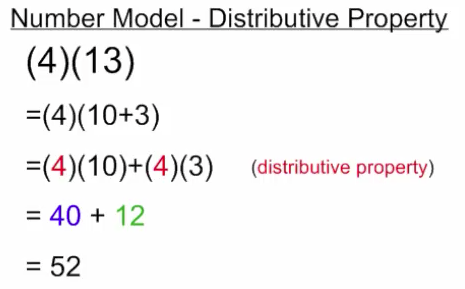2.5  – Numeracy – Multiplying - Distributive Property 2

Smart Notebook Lesson 2.5

Learning Goal:

By the end of this lesson I should be able to MULTIPLY double and triple digit positive numbers together using the DISTRIBUTIVE PROPERTY of MULTIPLICATION; WITHOUT the use of a calculator (technology).

Techniques:   Area Model and Distributive Property

Examples:

(a)                18 x 25

(b)               28 x 85

(c)                24 x 15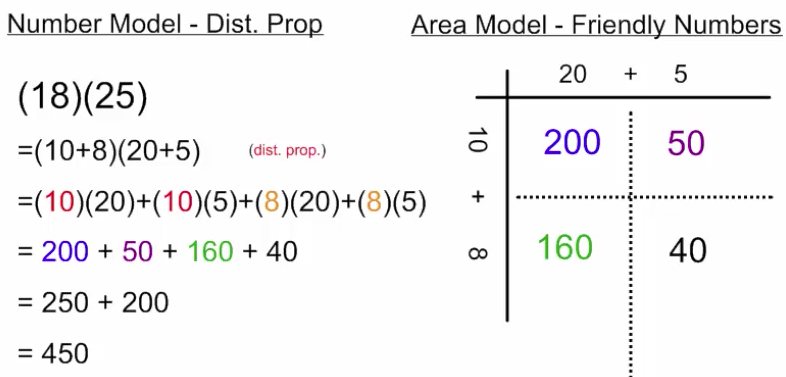2.6  – Numeracy – Multiplying - Column Model

Smart Notebook Lesson 2.6

Learning Goal:

By the end of this lesson I should be able to MULTIPLY double and triple digit positive numbers together using a COLUMN MODEL; WITHOUT the use of a calculator (technology).

Techniques:   Area Model and Column Model

Examples:

(a)                13 x 4

(b)               25 x 18

(c)                85 x 28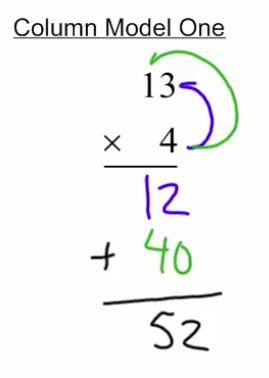2.7  – Numeracy – Multiplying - Column Model 2

Smart Notebook Lesson 2.7

Learning Goal:

By the end of this lesson I should be able to MULTIPLY double and triple digit positive numbers together using a COLUMN MODEL; WITHOUT the use of a calculator (technology).

Techniques:   Area Model and Column Model

Examples:

(a)                12 x 45

(b)               125 x 24

(c)                435 x 124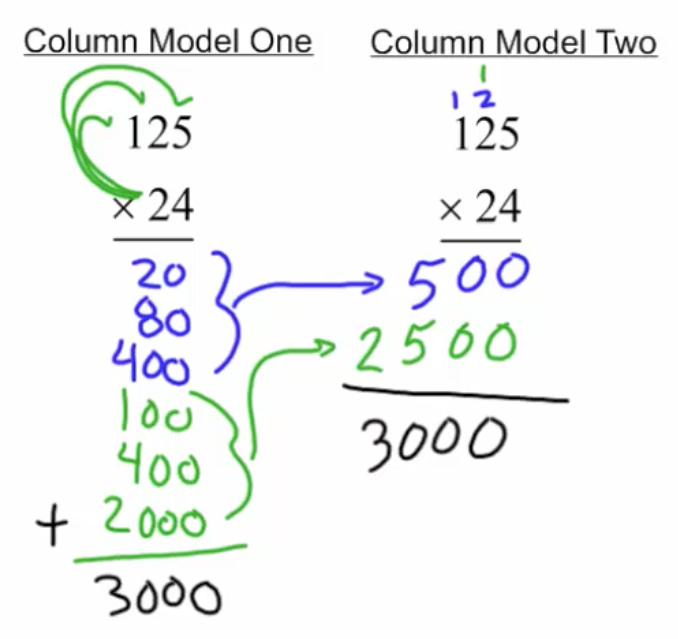2.8  – Numeracy – Multiplying - One Decimal Place

Smart Notebook Lesson 2.8

Learning Goal:

By the end of this lesson I should be able to MULTIPLY together a positive number and a number with ONE or TWO decimal places, WITHOUT the use of a calculator (technology).

• Multiplying by a decimal that is less than one, product should get smaller. (6)(0.2) = 1.2

Techniques:   Area Model and Column Model

Examples:

(a)                (7)(0.1)

(b)               (4)(0.6)

(c)                (5)(0.9)

(d)                (3)(0.75)2.9  – Numeracy – Multiplying - Two Decimal Places

Smart Notebook Lesson 2.9

Learning Goal:

By the end of this lesson I should be able to MULTIPLY together two decimal numbers (tenths), WITHOUT the use of a calculator (technology).

• Multiplying by a decimal that is less than one, product should get smaller. (6)(0.2) = 1.2

• Multiplying by a decimal that is larger than one, product should get larger. (6)(1.2) = 7.2

Techniques:   Area Model and Column Model

Examples:

(a)                (3)(4.1)

(b)               (4.5)(1.5)

(c)                (6.2)(1.5)2.10  – Numeracy – Multiplying - Two Decimal Places 2

Smart Notebook Lesson 2.10

Learning Goal:

By the end of this lesson I should be able to MULTIPLY together two decimal numbers (hundredths), WITHOUT the use of a calculator (technology).

Techniques:   Column Model

Examples:

(a)                (6.5)(1.5)

(b)               (3.52)(2.4)

(c)                (6.78)(3.45)2.11  – Numeracy – Multiplying - Positive number times a negative number

Smart Notebook Lesson 2.11

Learning Goal:

By the end of this lesson I should be able to Multiply a positive number and a negative number together, WITHOUT the use of a calculator (technology).

• When we multiply a positive number and a negative number together, the final product (result) ends up being a negative value.
• (+) x (-) = (-)

Techniques:   Number Model and Area Model

Examples:

(a)                (3)(-4)

(b)               (9)(-8)

(c)                (48)(-125)2.12  – Numeracy – Multiplying - Negative number times a negative number

Smart Notebook Lesson 2.12

Learning Goal:

By the end of this lesson I should be able to Multiply a negative number and a negative number together, WITHOUT the use of a calculator (technology).

• When we multiply a positive number and a negative number together, the final product (result) ends up being a negative value.
• (+) x (-) = (-)
• When we multiply a negative number and a negative number together, the final product (result) ends up being a negative value.
• (-) x (-) = (+)
• An ''odd'' number of negative signs in a multiplication or division problem leads to a negative answer.
• An ''even'' number of negative signs in a multiplication or division problem lead to a positive answer.

Techniques:   Number Model and Area Model

Examples:

(a)                (-9)(-8)

(b)               (4)(-9)(-3)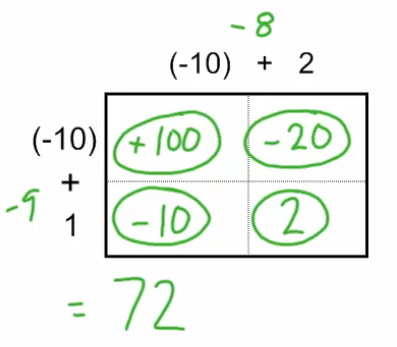2.13  – Numeracy – Multiplying - Area Model revisited

Smart Notebook Lesson 2.13

Learning Goal:

By the end of this lesson I should be able to Multiply positive and negative numbers together using an Area Model, WITHOUT the use of a calculator (technology).

Techniques:  Area Model

Examples:

(a)                (-9)(-8)

(b)               (98)(18)

(c)                (66)(-25)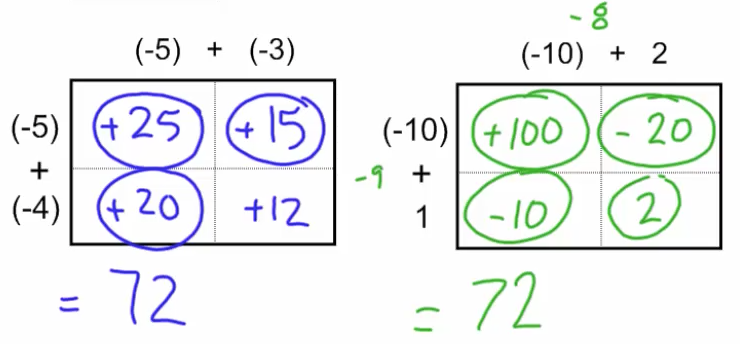2.14  – 2.15 - Numeracy – Multiplying - Review and Final Assessment

Task 2.14 – Review - Word

Task 2.14 - Review - Pdf

Final Assessment 2.15 - Word

Final Assessment 2.15 - Pdf

Summative Activities for the Multiplying Unit